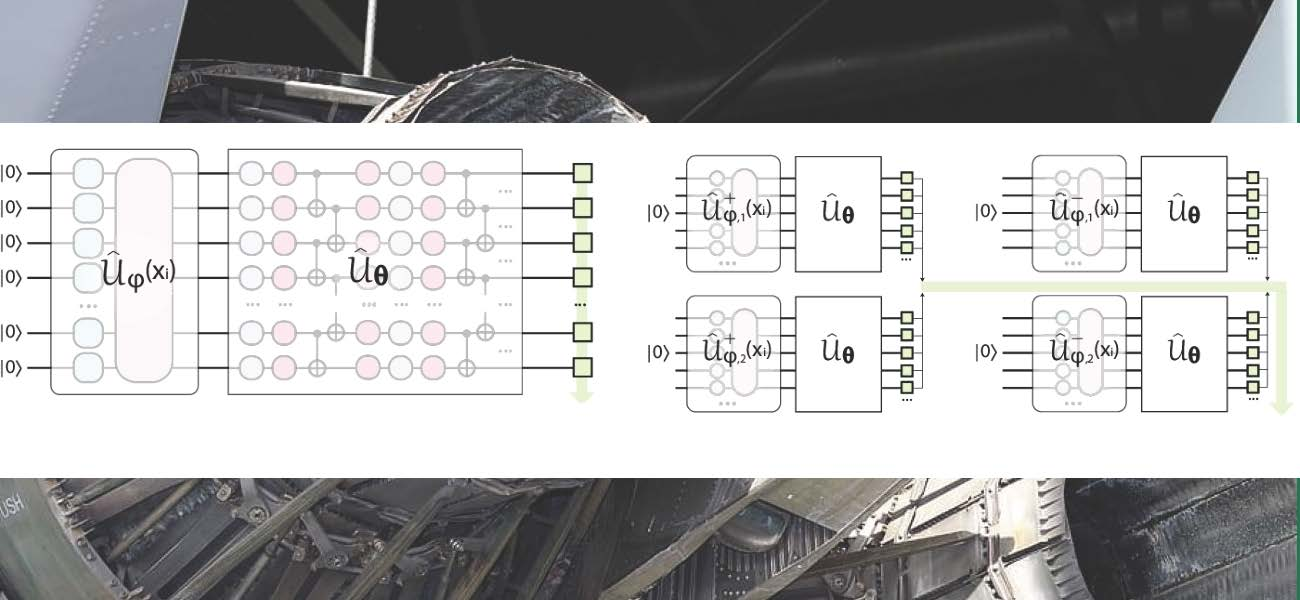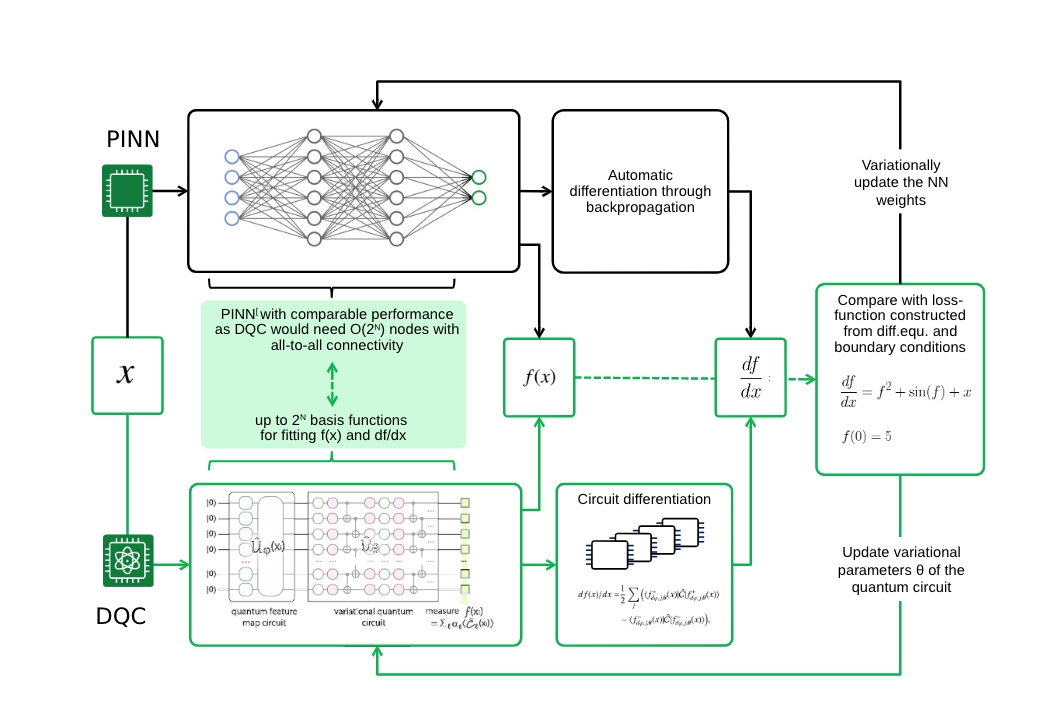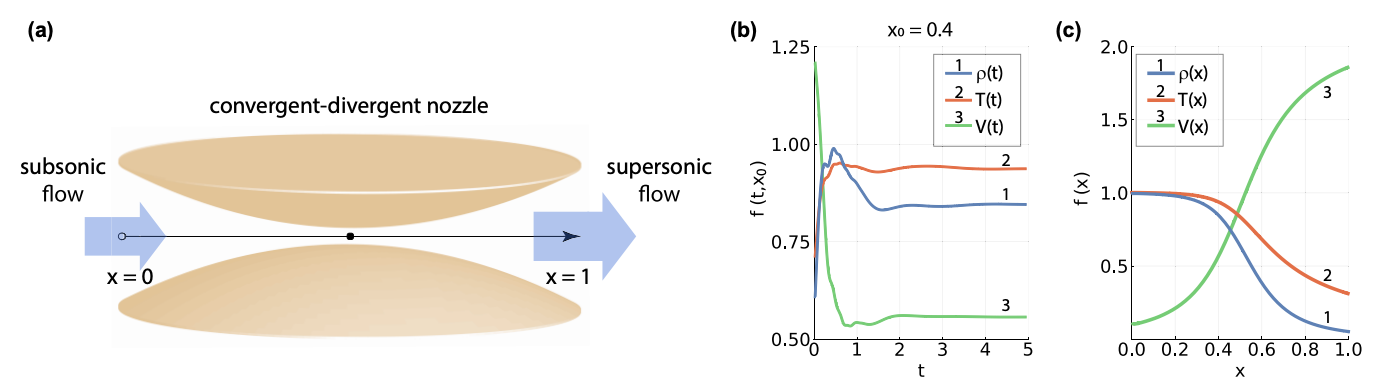# The power of differentiable quantum circuits

,

May 11, 2022Differential equations play central roles across a wide variety of disciplines and solving them often pushes classical hardware to its limits. Thus, it is natural to wonder whether quantum computers could help solve these types of problems. Most attempts at implementing differential equation solvers in quantum computers have severe shortcomings. These are especially acute when one considers the limitations of today’s noise- and size-limited quantum hardware. Most quantum solvers require deep and complex quantum circuits more suitable for quantum computers that will take many years to develop.

At PASQAL, we propose an alternative approach suitable for today’s quantum computers. Our method is inspired by a new paradigm for solving differential equations known as physics-informed machine learning (PIML). If you are not familiar with this approach, we recommend you read our post on this subject before moving on.

Done? Let’s continue then!

## Introducing differentiable quantum circuits

As you recall, PIML uses a universal function approximator (UFA) to represent solutions to differential equations. A good UFA has to satisfy two conditions. It must have large ‘expressivity’ to approximate solutions for a wide range of problems and it must be efficiently differentiable with respect to the input and the tunable parameters. The former derivative is needed to evaluate the UFA in the differential equation, the latter to train the model.

Expressivity is one of quantum computers’ strengths as they have access to an exponentially large state space. Thus, we pondered on whether quantum computers could be used as UFA generators. But, the question of differentiability loomed large. PASQAL’s team found a solution to this challenge by introducing the notion of Differentiable Quantum Circuit (DQC). DQCs are highly expressive UFAs whose derivatives can be calculated analytically. The latter is critical to implementing the routine on noisy near-term quantum hardware, as numerical differentiation on such devices is unreliable.

DQCs can thus be used as part of PIML solvers boosted by the rich expressivity of quantum computers.

## Quantum expressivity

Before we move on, we wish to shed some light on the notion of expressivity. In general, any function can be approximated using a sum of basis functions like Fourier series’ periodic functions or Chebyshev polynomials. The more terms we can add to the sum, the wider the variety of functions we can approximate. In other words, every additional term makes the sum more expressive.

So why do we say that DQCs have high expressive power? A DQC is a quantum state whose average energy can be used as a UFA. A DQC uses a quantum computer and involves two key elements: a quantum feature map followed by a tunable variational circuit (Figure 1). These steps are implementable in present-day quantum hardware. First, the feature map generates basis functions by encoding the dependence on the input variables into the rotation angles of single-qubit gates. Then, effectively the variational circuit generates combinations of these basis functions.

The critical point is that the number of possible combinations in-principle grows exponentially with the number of qubits. Tapping into that resource is key for DQC’s expressivity.

## Automatic differentiation

Up until now, we have learned how to build highly expressive and tunable functions using a quantum computer. That doesn’t mean these functions are UFAs, though. UFAs must be trainable in the PIML sense. This means that they must be differentiable with respect to the input parameters and the tunable parameters. Derivatives are traditionally calculated numerically using some variant of the finite difference method. However, quantum computers are far too noisy to do so reliably. This is not a problem for DQCs as all its derivatives can be calculated analytically and without sampling the entire quantum state.

This makes DQC ideal for running on near-term quantum hardware, especially on PASQAL’s neutral atom quantum computers, as we explain in another post.DQC brings the PIML workflow to the quantum realm. In DQC, instead of using a Physics-informed neural network (PINN) as the UFA, we use a quantum neural network of a specific type, known as a differentiable quantum circuit.

## Modeling supersonic flow

As a use-case example, we used the DQC method to solve a paradigmatic task in the aerospace industry. Namely, solving the Navier-Stokes equations for a viscous fluid in a convergent-divergent nozzle setup (Figure 2a). Of particular interest is the case of subsonic-supersonic transitions, where flow velocity breaks the sound barrier after the center of the nozzle. The qualitative behavior of state variables is known. For instance, the temperature and density drop after passing the nozzle. However, obtaining quantitative results is challenging. We tried the DQC algorithm on this problem using a quantum emulator. And the results were excellent, with the solver accurately describing the physics of the subsonic-supersonic transition (Figure 2b).(a) We consider an example of fluid dynamics problem corresponding to a convergent-divergent nozzle.The air flows from converging part of the nozzle (x < 0.5), passes through the throat placed symmetrically in the middle, and exits to diverging part (x > 0.5). (b) System variables [density ρ(t), temperature T(t), and velocity V(t)] are shown as functions of time, near the centre of the nozzle at x0 = 0.4. For clarity, we label the corresponding curves by 1, 2, and 3. © Steady state solutions are plotted as functions of the spatial dimension x, with the same labelling.

Next, we are moving towards real quantum hardware in PASQAL, and considering more complex use cases. Stay tuned!

The main contributing authors to this blog are Evan Philip, Mario Dagrada, and Vincent Elfving.